# Samacheer Kalvi 6th Maths Solutions Term 3 Chapter 1 Fractions Ex 1.1## Tamilnadu Samacheer Kalvi 6th Maths Solutions Term 3 Chapter 1 Fractions Ex 1.1

Question 1.
Fill in the blanks.
(i) 7$$\frac{3}{4}$$ + 6$$\frac{1}{2}$$ = _______
(ii) The sum of whole number and a proper fraction is called ______
(iii) 5$$\frac{1}{3}$$ – 3$$\frac{1}{2}$$ = ______
(iv) 8 ÷ $$\frac{1}{2}$$ = ______
(v) The number which has its own reciprocal is _______.
Solution:
(i) 14$$\frac{1}{4}$$
(ii) Mixed Fraction
(iii) 1$$\frac{5}{6}$$
(iv) 16
(v) 1

Question 2.
Say True or False
(i) 3$$\frac{1}{2}$$ can be written as 3 + $$\frac{1}{2}$$.
(ii) The sum of any two proper fractions is always an improper fraction.
(iii) The mixed fraction of $$\frac{13}{4}$$ is 3$$\frac{1}{4}$$.
(iv) The reciprocal of an improper fraction is always a proper fraction.
(v) 3$$\frac{1}{4}$$ × 3$$\frac{1}{4}$$ = 9$$\frac{1}{16}$$
Solution:
(i) True
(ii) False
(iii) True
(iv) True
(v) FalseQuestion 3.
Solution:
(i) Find the sum of $$\frac{1}{7}$$ and $$\frac{3}{9}$$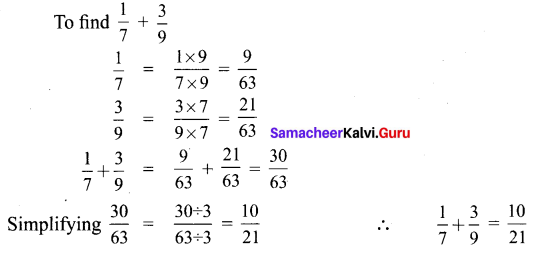(ii) What is the total of 3$$\frac{1}{3}$$ and 4$$\frac{1}{6}$$.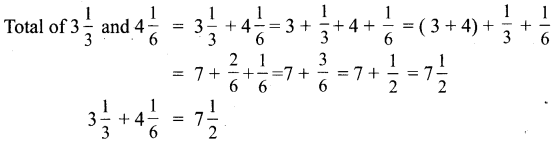(iii) Simplify : 1$$\frac{3}{5}$$ + 5$$\frac{4}{7}$$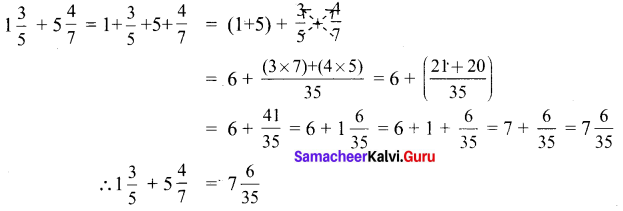(iv) Find the difference between $$\frac{8}{9}$$ and $$\frac{2}{7}$$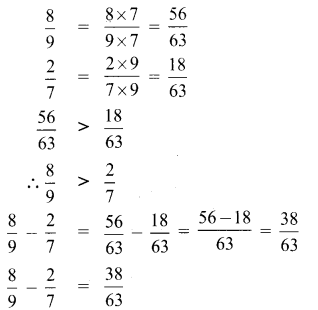(v) Subtract 1$$\frac{3}{5}$$ and 2$$\frac{1}{3}$$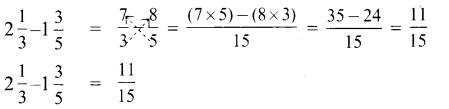(vi) Simplify: 7$$\frac{2}{7}$$ – 3$$\frac{4}{21}$$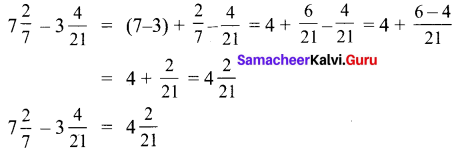Question 4.
Convert mixed fraction into improper fractions and vice versa: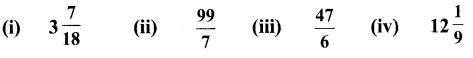Solution: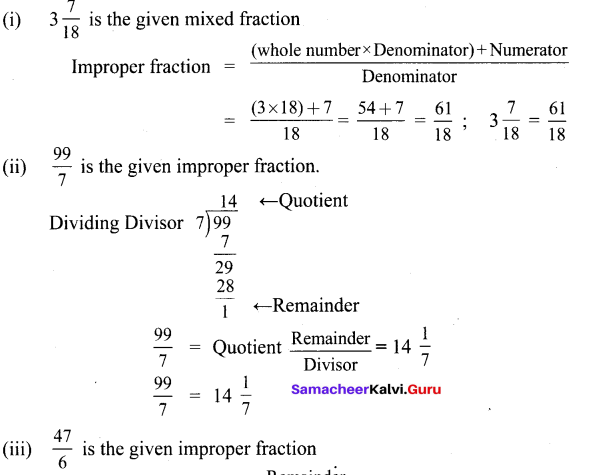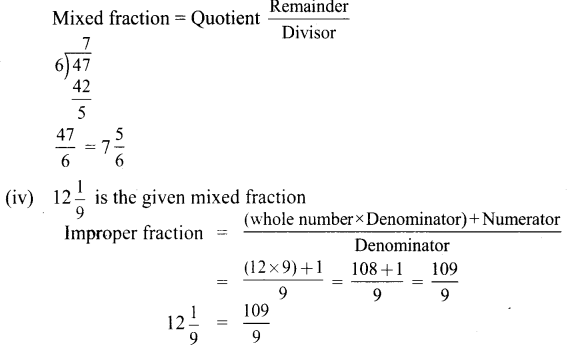Question 5.
Multiply the following :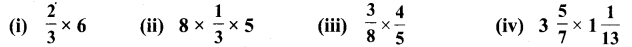Solution: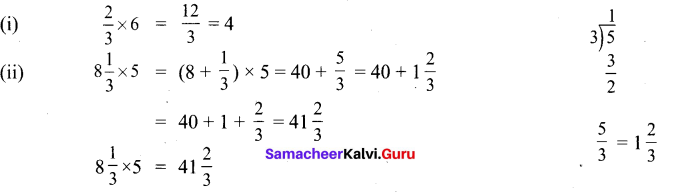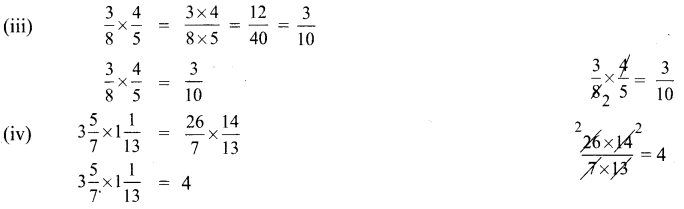Question 6.
Divide the following: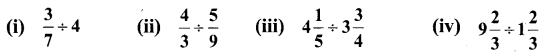Solution: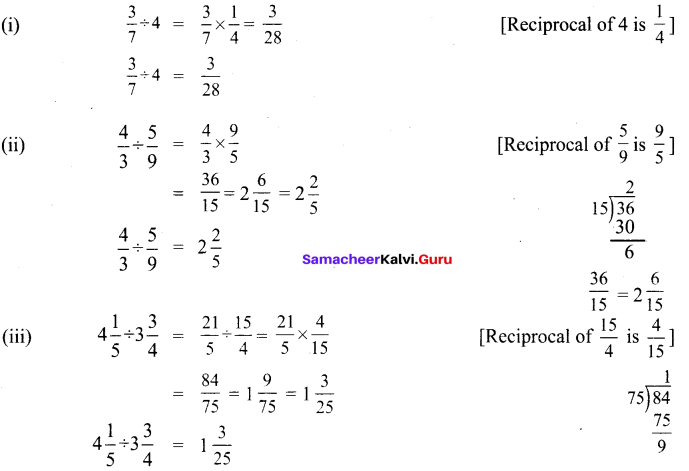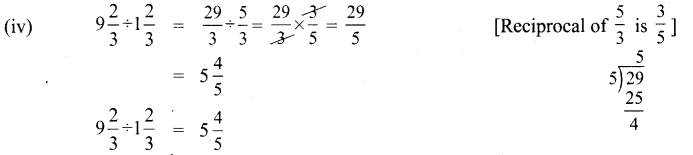Question 7.
Gowri purchased 3$$\frac{1}{2}$$ kg of tomatoes, $$\frac{3}{4}$$ kg of brinjal and 1$$\frac{1}{4}$$ kg of onion, what is the total weight of the vegetables she bought?
Solution:
Weight of tomatoes Gowri purchased = 3$$\frac{1}{2}$$ kg
Weight of Brinjal purchased = $$\frac{3}{4}$$ kg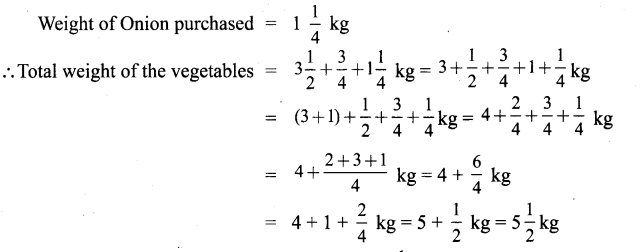Total weight of vegetables that Gowri purchased = 5$$\frac{1}{2}$$ kgQuestion 8.
An oil tin contains 3$$\frac{3}{4}$$ litres of oil of which 2$$\frac{1}{2}$$ litres of oil is used. How much oil is left over?
Solution: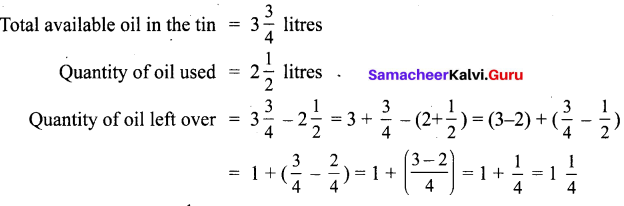Quantity of oil leftover = 1$$\frac{1}{4}$$ litres.

Question 9.
Nilavan can walk 4$$\frac{1}{2}$$km in an hour. How much distance will he cover in 3$$\frac{1}{2}$$ hours?
Solution:
Distance walked by Nilavan in one hour = 4$$\frac{1}{2}$$ km.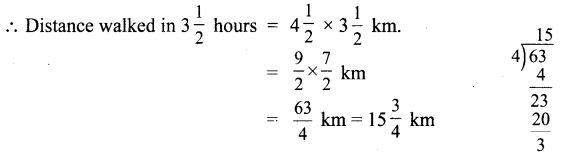Nilavan walks 15$$\frac{3}{4}$$ km in 3$$\frac{1}{2}$$ hoursQuestion 10.
Ravi bought a curtain of length 15$$\frac{3}{4}$$ m. If he cut the curtain into small pieces each of length 2$$\frac{1}{4}$$ m, then how many small curtains will he get?
Solution: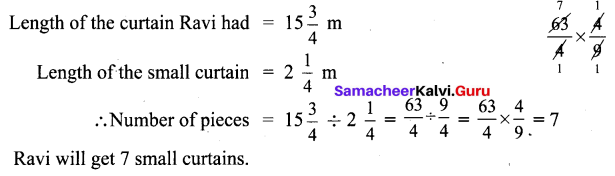Objective Type Questions

Question 11.
Whcih of the following statement is incorrect?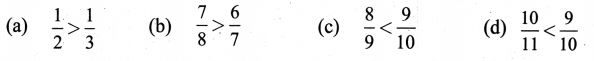Solution:
(d)$$\frac{10}{11}<\frac{9}{10}$$
Hint: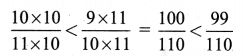Question 12.
The difference between $$\frac{3}{7}$$ and $$\frac{2}{7}$$ is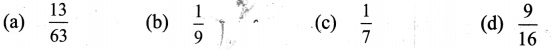Solution:
(a) $$\frac{13}{63}$$
Hint: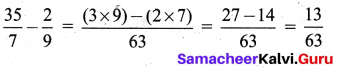Question 13.
The reciprocal of $$\frac{53}{17}$$ is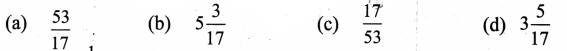Solution:
(c) $$\frac{17}{53}$$
Hint:
$$\frac{\frac{1}{53}}{\frac{53}{17}}=\frac{17}{53}$$Question 14.
If $$\frac{6}{7}$$ = $$\frac{A}{49}$$, then the value of A is
(a) 42
(b) 36
(c) 25
(d) 48
Solution:
(a) 42

Question 15.
Pugazh has been given four choices for his pocket money by his father. Which of the choices should he take in order to get the maximum money?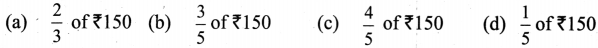Solution:
(c) $$\frac{4}{5}$$ of ₹150